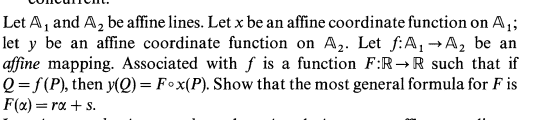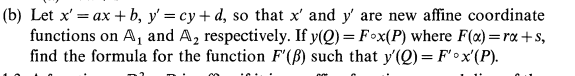# Mapping beetween affine coordinate functions

• Aleoa

## Homework Statement## Homework Equations

As the book says , an affine function of a line is $$A\rightarrow \mathbb{R}$$ and represent the real number that, multiplied for a basis and starting from an origin of the line gives a certain point of the line, so a origin of the line and a basis is implicitly taken when defining the affine coordinate function.

## The Attempt at a Solution

[/B]
Since for each of the 2 affine lines, the affine coordinate function is simply a number that is multiplied to the basis, what i think is that $$f(\alpha) = r\alpha$$. But this seems not to be correct. Why ?

#### Attachments

Suppose we have 2 affine lines (NOT paralells), for each line we set an arbitrary origin and an arbitrary basis. I choose a point P in the first line and i get x(P) = 3. Then, I choose an arbitrary point P' in the second line , and i get y(P') = 1 . So, the mapping beetween the first line and the second line is F(α)=α/3.

Why it's wrong and F(α) should be F(α)=rα+s, and not just F(α)=rα as my result ?

Well I think your result is not wrong, but by making ##F(\alpha) = r\alpha##, you're assuming that the base of one line is getting mapped into the base of the other line, that is, zero coordinate is mapped into zero coordinate. And that doesn't necessarily always hold, so you have the constant ##s## that is essentially base coordinate translation. I think it works that way, that's why ##F(\alpha) = r\alpha + s## is the most general form.

But, the two affine coordinate functions x and y ( which are already defined), haven't implicitely fixed a basis and an origin for the first line and a basis and an origin for the second line?
This means that, if a want to change the basis and the origin of x or y, i can modifify the definition of x or y.
Am i wrong ?

Well, x and y functions assign real numbers to points on two lines, and F is basically a real function that maps coordinates of points on one line to coordinates of points on the other line. So, definitely, F doesn't have to map zero to zero, because you can choose it to map the origin of a line into a point that isn't the origin of the other line. So what they're asking for in the exercise is like the general form of this function. What you're saying when you're implying your form is that ##s## in ##F=r\alpha + s## is always zero, and I'm saying that it's only zero if your zero coordinate(and that's the base of the line since coordinates are defined as multiples of a basis) is mapped into the zero coordinate of the other line. That is, in your formula, your function f always maps base of the line into base of the other line, and I'm saying it doesn't have to.

And yes, changing basis means changing x or y coordinates of points along those lines, while changing base is really a geometrical thing that corresponds to translation along the line by some constant parameter. However, function F will be numerically different if you change base or basis of those lines, but it's general form ##r\alpha + s## will be preserved, I think, just ##r## and ##s## will be different constants.

•Aleoa
This exercise has a part 2.$F'$ should be a linear function such that $F'(x) = \frac{c}{a}y$ and $F'(b) = d$.
However , i don't know how to write a formula for $F'$ ...

#### Attachments

Hmm, you're making the same type of mistake I think, you're assuming x=0 is being mapped into y=0.

This F function basically maps x into y, so you can say
$$y=rx+s$$
That's the old function F. Now transformed one does the same thing with x' and y'. So it should also be linear, since your change of basis transformations are linear, but constants r' and s' will be different. So you can assume
$$F'(\alpha)=r'\alpha+s'$$ and you want to derive the new constants.
So to correct your assumption, I'd say, ##x=0## is mapped by F into ##y=s##, using formula for F. That means that ##x'=b## is mapped by F' into ##y'=cs+d##, because same point is mapped into same point, we just changed the coordinates. Hope that helps some.

•Aleoa
Thanks so much. Now, it's all clear :)

•Antarres